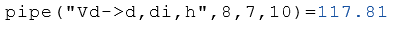# Class Pipe

Calculation of the properties of hollow cylinders

## Description

The class Pipe calculates properties of a hollow cylinder.

## Properties

The following properties can be queried as a result or passed as an argument.

#### Description

Längen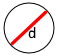d Outside diameter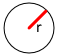r Outside radius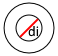di Inside diameter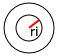ri Inside radius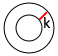k Donut width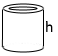h Height of the Cylinder
Umfang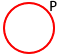P Outside perimeter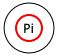Pi Inside perimeter
Basis-Flächen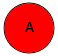A Total area of the circle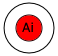Ai Inside area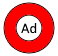Ad Area of the donut
Kreisring Oberflächen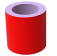Sd Outside surefaceSi Inside sureface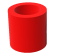S Total sureface include top and base
Volumen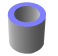Vd Volume of the donutVi Inside volume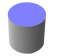V Total volume

## Syntax

Object = Pipe ("arg names", arguments)

Value = Pipe ("get -> arg names", arguments)

## Example

Calculation of the properties with the outer diameter $$d$$ the inner diameter $$di$$ and the height $$h$$.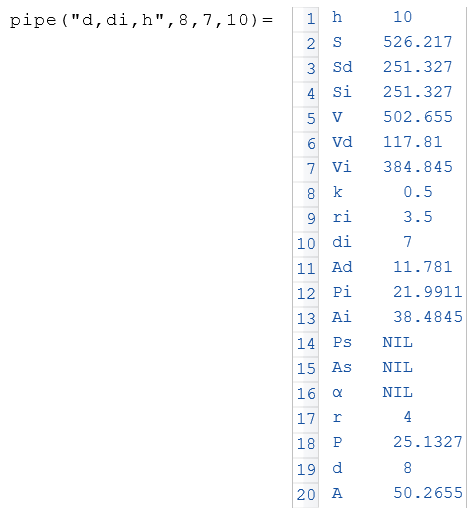The following example displays the volume $$V$$ from the object $$a$$.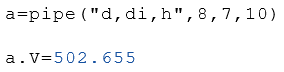Calculation of the volume $$Vd$$ (volume of the ring).